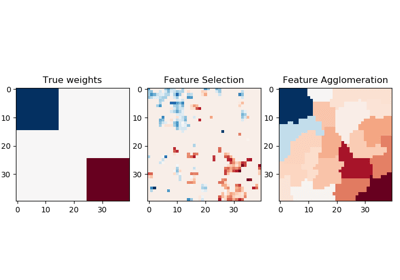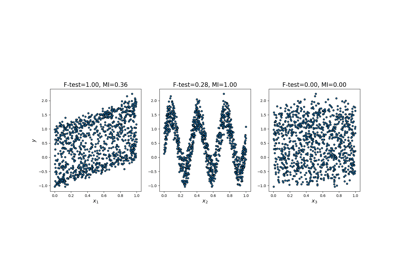/scikit-learn

# sklearn.feature_selection.f_regression

`sklearn.feature_selection.f_regression(X, y, center=True)` [source]

Univariate linear regression tests.

Linear model for testing the individual effect of each of many regressors. This is a scoring function to be used in a feature selection procedure, not a free standing feature selection procedure.

This is done in 2 steps:

1. The correlation between each regressor and the target is computed, that is, ((X[:, i] - mean(X[:, i])) * (y - mean_y)) / (std(X[:, i]) * std(y)).
2. It is converted to an F score then to a p-value.

For more on usage see the User Guide.

Parameters: `X : {array-like, sparse matrix} shape = (n_samples, n_features)` The set of regressors that will be tested sequentially. `y : array of shape(n_samples).` The data matrix `center : True, bool,` If true, X and y will be centered. `F : array, shape=(n_features,)` F values of features. `pval : array, shape=(n_features,)` p-values of F-scores.

`mutual_info_regression`
Mutual information for a continuous target.
`f_classif`
ANOVA F-value between label/feature for classification tasks.
`chi2`
Chi-squared stats of non-negative features for classification tasks.
`SelectKBest`
Select features based on the k highest scores.
`SelectFpr`
Select features based on a false positive rate test.
`SelectFdr`
Select features based on an estimated false discovery rate.
`SelectFwe`
Select features based on family-wise error rate.
`SelectPercentile`
Select features based on percentile of the highest scores.

## Examples using `sklearn.feature_selection.f_regression`Feature agglomeration vs. univariate selectionComparison of F-test and mutual informationPipeline Anova SVM

© 2007–2018 The scikit-learn developers